# Estimating Multivariate Functions and Derivatives using Local Polynomial Regression in Matlab

## 1. Theoretical Background

I want to start with some theory which will in the end lead us to the Matlab coding. If you are not interested how to derive the estimator in detail, feel free to skip this section. If you are interested in a detailed discussion about local polynomial regression I recommend to have a look into Fan and Gijbles (1996).

We assume that a smooth curveis observed at independent randomly-distributed pointscontaminated with additional noise. Our model is then given by

(1)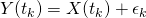where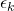are i.i.d. random variables with,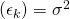andis independent of.

Our goal is then to estimate partial derivatives  which are denoted using a vector(2)For the following a little notation is needed. For any vectorswe define,. Let,and consider a multivariate local polynomial estimator of order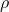for a pointgiven bythat solves

(3)is any non-negative, symmetric and bounded  multivariate kernel function andabandwidth matrix. For simplicity, we assume thathas main diagonal entriesand zero elsewhere. Remember thatis a vector of size, the desired estimates are then given bywhere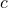is the position in the vector which is dependent on the ordering of the polynomials in (3).

To code the estimator in Matlab we will write down the estimator using matrices. Let,using this notation together with, (3) can then be rewritten as

(4)(4) looks very much like a least squares problem, and under some regularity assumptions the solution is given by

(5)This expression is then indeed easy to code.

## 2. Implementation using Matlab

The implementation is based on product kernels of a Gaussian and an Epanechnikov kernel. For running a kernel regression on very large data sets bounded kernels like the Epanechnikov kernel are of particular interest because a lot of entries in (4) will become zero ifis far from. A conscientious programmer will thus chose only a certain window based onand bandwidthof observations to construct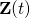and compute to (5). Since I am not a conscientious programmer and I chose to keep the implementation simple this time, in this implementation this speed-up opportunity is omitted 😉

% ------------------------------------------------------------------------------
% Description: g-Dimensional local polynomial estimator
%
% ------------------------------------------------------------------------------
% Usage:       -
% ------------------------------------------------------------------------------
% Inputs Simulation:
%X - g dimensional inputcoordinate (Dimension: Txg)
%Y - function value (Dimension: Tx1)
%x - g dimensional output coordinates (Dimension: T_outxg)
%b - g dimensional vector of  bandwiths (Dimension: 1xg)
%p - degree of polynomial (rho)

% ------------------------------------------------------------------------------
%Output:
%fit  - Smoothed curves and derivatives

% ------------------------------------------------------------------------------
% Keywords:    local polynomial surface estimator, derivatives
% ------------------------------------------------------------------------------
% ------------------------------------------------------------------------------
% Author:      Heiko Wagner, 2017/01/18
% ------------------------------------------------------------------------------

function [fit] = multilocpoly(X,Y,x,b,p,kernel)

%% Epanechnikov Kernel function
function [k]=epan(t);
%t=(-100:100)/50
k= 3/4*(1-t.^2);
k((abs(t)-1)>0)=0;
end

g= size(X,2) ;
T = size(X,1);
T_out = size(x,1);
dummy=permn(0:p,g);             %%%Get all possible combinations
k=dummy( sum(dummy,2)<p+1,:);
pp=size(k,1);
Z = zeros( T, pp );

function [beta_j]=getpoint(x_j)
%%%Construct g dimensional grid around point j, at this pint you can improve the code if you use the Epanechnikov Kernel by only selecting certain observations
Xn = X -  repmat( x_j,T,1 );

%%%Construct polynomials
for m=1:pp
Z(:,m)= prod( Xn.^repmat(k(m,:),T,1) ,2);
end

%%%Construct g-dimensional product kernel
A=1;
if(strcmp(kernel,'Gauss')==1)   %Use Gaussian Kernel
for (m=1:g)
A= A.*normpdf(Xn(:,m)/b(m))/b(m)  ;
end
else                            %Use Epanechnikov Kernel
for (m=1:g)
A= A.*epan(Xn(:,m)/b(m))/b(m)  ;
end
end
A=diag(A);

%%%Solve for beta_j
beta_j = inv(Z' * A * Z) * Z' * A * Y;
end
%%%To estimate not just a single point we estimate the function for an entire set of g dimensional output coordinates (x)
[out]=arrayfun(@(s) getpoint( x(s,:) ), 1:T_out , 'UniformOutput', false);
fit=[out{:}]'*diag(max(1,factorial( sum(k,2) )));
end
end

This site uses Akismet to reduce spam. Learn how your comment data is processed.